IMO Shortlist 2010 problem G7

Kvaliteta:
Avg: 0,0
Težina:
Avg: 9,0
Three circular arcs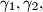$\gamma_1, \gamma_2,$ and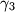$\gamma_3$ connect the points$A$ and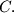$C.$ These arcs lie in the same half-plane defined by line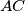$AC$ in such a way that arc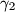$\gamma_2$ lies between the arcs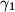$\gamma_1$ and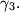$\gamma_3.$ Point$B$ lies on the segment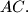$AC.$ Let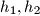$h_1, h_2$, and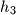$h_3$ be three rays starting at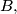$B,$ lying in the same half-plane,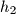$h_2$ being between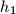$h_1$ and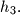$h_3.$ For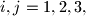$i, j = 1, 2, 3,$ denote by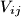$V_{ij}$ the point of intersection of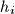$h_i$ and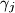$\gamma_j$ (see the Figure below). Denote by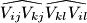$\widehat{V_{ij}V_{kj}}\widehat{V_{kl}V_{il}}$ the curved quadrilateral, whose sides are the segments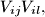$V_{ij}V_{il},$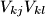$V_{kj}V_{kl}$ and arcs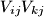$V_{ij}V_{kj}$ and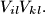$V_{il}V_{kl}.$ We say that this quadrilateral is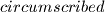$circumscribed$ if there exists a circle touching these two segments and two arcs. Prove that if the curved quadrilaterals {{ INVALID LATEX }} are circumscribed, then the curved quadrilateral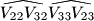$\widehat{V_{22}V_{32}}\widehat{V_{33}V_{23}}$ is circumscribed, too.

Proposed by Géza Kós, Hungary
Izvor: Međunarodna matematička olimpijada, shortlist 2010